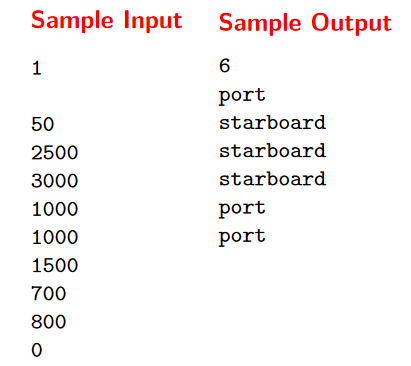## Problem Statement

Before bridges were common, ferries were used to transport cars across rivers. River ferries, unlike their larger cousins, run on a guide line and are powered by the river’s current.

Two lanes of cars drive onto the ferry from one end, the ferry crosses the river, and the cars exit from the other end of the ferry.

The cars waiting to board the ferry form a single queue, and the operator directs each car in turn to drive onto the port (left) or starboard (right) lane of the ferry so as to balance the load. Each car in the queue has a different length, which the operator estimates by inspecting the queue. Based on this inspection, the operator decides which side of the ferry each car should board, and boards as many cars as possible from the queue, subject to the length limit of the ferry.

Your job is to write a program that will tell the operator which car to load on which side so as to maximize the number of cars loaded.

## Input

The input begins with a single positive integer on a line by itself indicating the number of the cases following, each of them as described below. This line is followed by a blank line, and there is also a blank line between two consecutive inputs. The first line of input contains a single integer between 1 and 100: the length of the ferry (in metres). For each car in the queue there is an additional line of input specifying the length of the car (in cm, an integer between 100 and 3000 inclusive). A final line of input contains the integer 0. The cars must be loaded in order, subject to the constraint that the total length of cars on either side does not exceed the length of the ferry. Subject to this constraint as many cars should be loaded as possible, starting with the first car in the queue and loading cars in order until no more can be loaded.

## Output

For each test case, the output must follow the description below. The outputs of two consecutive cases will be separated by a blank line. The first line of output should give the number of cars that can be loaded onto the ferry. For each car that can be loaded onto the ferry, in the order the cars appear in the input, output a line containing ‘port’ if the car is to be directed to the port side and ‘starboard’ if the car is to be directed to the starboard side. If several arrangements of the cars meet the criteria above, any one will do.

```				```
from sys import stdin

PORT = "port"
STARBOARD = "starboard"

# Ferry length in cm

# Read car dimmensions until 0 is reached
cars = []

while car>0:
cars.append(car)

return length, cars

def best_dist(ferry_length, cars):

csum =
for c in cars:
csum.append(c+csum[-1])

# Using dicts instead of lists because they're faster for sparse matrices
dp = [dict() for _ in range(len(cars)+1)]
dp = True
side = [dict() for _ in range(len(cars)+1)]

last_car = 0
for i, car in enumerate(cars, 1):
for j in dp[i-1].keys():
# Load car into port side if there is enough space
if ferry_length-(csum[i-1]-j) >= car:
dp[i][j] = True
side[i][j] = PORT
last_car = i

# Load car starboard side if there is enough space
if ferry_length-j>=car:
dp[i][j+car] = True
side[i][j+car] = STARBOARD
last_car = i

# Select one of the valid combinations (any will do)
last_length = list(dp[last_car].keys())

# Recostruct selected combination from last to first
sides_chosen = []
while (last_length in side[last_car]):
s = side[last_car][last_length]
sides_chosen.append(s)
if s == STARBOARD:
last_length -= cars[last_car-1]
last_car -= 1

return list(reversed(sides_chosen))

if __name__ == '__main__':

for c in range(ncases):